The KRIGE2D Procedure

Anisotropic Models

Subsections:

In all of the theoretical models considered previously, the lag distance h is entered as a scalar value. This implies that the correlation between the spatial process at two point pairs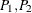is dependent only on the separation distance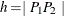, not on the orientation of the vector. A spatial process described by an SRF {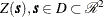} with this property is called isotropic, as is the associated covariance or semivariogram.

However, real spatial phenomena often show directional effects. Particularly in geologic applications, measurements along a particular direction might be highly correlated, while typically the perpendicular direction shows little or no correlation. Such processes are called anisotropic; see, for example, Journel and Huijbregts (1978, section III.B.4).

When the correlation structure varies across different directions, you need different models for each direction so that you can account correctly for the continuity within the SRF. The following subsections describe how techniques are applied to override the anisotropy effects for computational purposes. First, characteristics of anisotropy are examined.

The semivariogram sill is a measure of the process variability; hence the direction of the highest continuity is perpendicular to the direction where the highest sill occurs. If the sill is the same in all directions, then the direction with the highest range indicates highest continuity. The directions in which the spatial process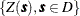is most and least correlated are called the major and minor axis of anisotropy, respectively.

In some cases, these directions are known a priori. This can occur in mining applications where the geology of a region is known in advance. In most cases however, nothing is known about possible anisotropy. Depending on the amount of data available, using several directions is usually sufficient to determine the presence of anisotropy and to find the approximate major and minor axis directions; see the discussion in the section Anisotropy in Chapter 109: The VARIOGRAM Procedure, documentation. You can find a detailed example of anisotropy investigation in the section An Anisotropic Case Study with Surface Trend in the Data in Chapter 109: The VARIOGRAM Procedure, documentation.

After you explore an anisotropic process and you identify the minor and major axis directions, you can compute the anisotropy factor parameter R which is defined as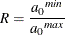where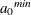is the semivariogram range in the direction of the minor axis and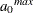is the semivariogram range in the direction of the major axis.

There are two types of anisotropy, depending on which semivariogram characteristics change in different directions. These types are the geometric and the zonal anisotropy, and either or both can be present. Both are examined in detail in the following subsections.